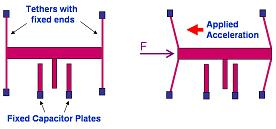Accelerometers

An accelerometer is a device that measures acceleration, or the rate of change of velocity with respect to time. Accelerometers come in various forms and sizes, but cutting edge micromachining technology advancements in recent years have allowed them to be built in microchip form.  Today, there are a multitude of semiconductor companies that manufacture accelerometer IC's that not only measure linear acceleration, but other parameters as well such as angular speed, vibrations, shock, and even tilt positions.

One of the pioneers in fabricating accelerometers in integrated circuit form is Analog Devices, which produces the ADXL50 accelerometer.  The ADXL50 provides an output voltage that varies proportionally with the amount of acceleration experienced along its sensitive axis. It has an input range of -50g to +50g, with a sensitivity of approximately 1 V per 50 g.  Thus, a 50-g acceleration would either decrease or increase the output at 0 g by 1V, depending on the direction of the acceleration.  Since the ADXL50 is calibrated to output 1.8V when there is no acceleration, the output would either 0.8 V or 2.8 V at 50 g, again depending on the acceleration's direction.

The ADXL50 is an example of a capacitive accelerometer, i.e., it measures capacitances in order to measure the acceleration. This accelerometer applies two basic principles of physics in its operation. The first one is Hooke's Law, which states that a spring, when stretched, will exert a restoring force F that's proportional to its increase in length x, i.e., F = kx. The second one is Newton's Second Law, which states that the force F exerted by a body is equal to its mass m multiplied by its acceleration a, i.e., F = mA.

Combining these two equations, A = kx/m, which means that a body with mass m will stretch a spring (whose elongation property is characterized by k) by a distance of x if its acceleration is A. The ADXL50 has a mass-spring system consisting of a bar of silicon (which is the mass) that is held by four tethers (one at each corner), as shown in Figure 1.

The four tethers, the feet of which are anchored, compose the spring system. When the mass is subjected to an acceleration, it moves with respect to the anchored feet of the tethers, causing the tethers to 'stretch' like a spring. The greater the acceleration experienced, the larger is the displacement. This system therefore translates the acceleration into a displacement, allowing the acceleration to be measured by measuring the displacement.Figure 1. A Differential Capacitive Accelerometer Mass-Spring

System at rest (left) and when subjected to acceleration (right)

The displacement of the bar is measured in terms of the difference between two capacitances formed by the accelerometer's structure in Figure 1.  The two fixed capacitor plates form a capacitor each with the inner capacitor plate that's attached to the moving mass, i.e., they both share a common capacitor plate (the one that moves with the mass).  The value of the capacitance of each capacitor changes with the movement of the inner capacitor.  Since the change in capacitance of one capacitor is opposite to that of the other capacitor, even the direction of the acceleration can be determined from the changes.

The amounts and rates of change of these two capacitances are then translated by on-chip signal conditioning circuits into an output voltage that indicates the strength and direction of the acceleration.  The on-chip signal conditioning circuitry may consist of amplifiers, filters, oscillators, demodulators, and even self-test circuitry.

Note that velocity is simply the integral of acceleration, and displacement is simply the integral of velocity.  As such, information about the velocity and displacement of the body may also be known by performing the necessary integration steps on the acceleration information obtained from the accelerometer.

Performance parameters for accelerometers include: 1) the Zero g Offset, or the voltage output at 0 g; 2) the Sensitivity, or the output voltage per g; 3) the Noise, which determines the minimum resolution of the sensor; 4) the Temperature Range; 5) the Bias Drift with Temperature, or how the 0 g output changes with temperature; 6) the Sensitivity Drift with Temperature, or how the 0 g output changes with temperature; 7) the Bandwidth; and 8) the Power Consumption.

Primary Reference: www.analog.com